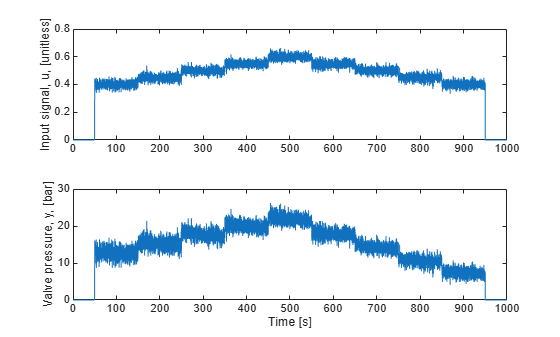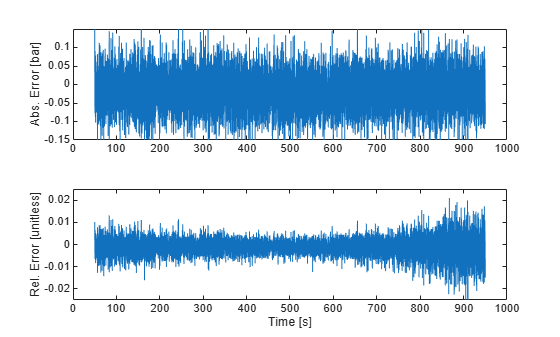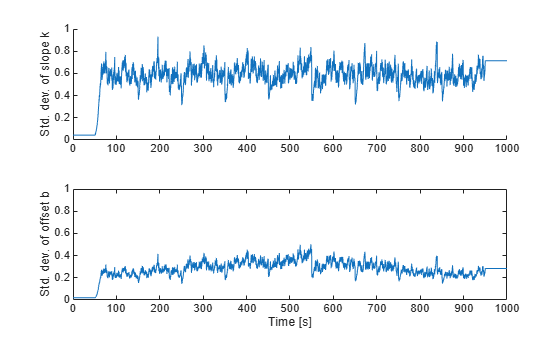# Line Fitting with Online Recursive Least Squares Estimation

This example shows how to perform online parameter estimation for line-fitting using recursive estimation algorithms at the MATLAB® command line. You capture the time-varying input-output behavior of the hydraulic valve of a continuously variable transmission.

### Physical System

The system is a continuously variable transmission (CVT) driven by a hydraulic valve, inspired by reference . The valve pressure is connected to the CVT which allows it to change its speed ratio and to transmit torque from the engine to the wheels. The input-output behavior of the valve can be approximated by:

`$y\left(t\right)=k\left(t\right)u\left(t\right)+b\left(t\right)\phantom{\rule{0.2777777777777778em}{0ex}}\phantom{\rule{0.2777777777777778em}{0ex}}for\phantom{\rule{0.2777777777777778em}{0ex}}\phantom{\rule{0.2777777777777778em}{0ex}}\frac{-b\left(t\right)}{k\left(t\right)}`

Here, t is the current time, y(t) is the valve pressure in bar, u(t) is the unitless input in the range of [0, 1]. The condition $\frac{-b}{k} is the dead-band of the valve.

The slope, k(t), and offset, b(t), depend on the system temperature. They vary as the system warms up from cold start to typical operating temperature. You want to estimate k(t) and b(t) based on noisy measurements of u(t) and y(t).

### Data

The true slope and offset parameters are k(0)=70 and b(0)=-15 at time t=0s. At t=50s the engine starts. The parameters vary over time until they reach k(950)=50 and b(950)=-13 at t=950s. The sample time is Ts=0.1s.

The content of the input signal u is critical for parameter estimation. Consider a case where u, and hence y, is constant. Then there are infinitely many k and b values that satisfy y = k u + b. u(t) must be persistently exciting the system for successful estimation of k(t) and b(t). In this example, the input u:

• is zero from t=0s until t=50s.

• has step changes to 0.40, 0.45, 0.50, 0.55, 0.60, 0.55, 0.50, 0.45, 0.40 every 100s, from t=50s until t=950s.

• a Gaussian random variable with zero mean, 0.02 standard deviation was added at each time step from t=50s until t=950s to provide extra excitation of system dynamics for identification purposes.

The output is generated with the aforementioned true values of k(t), b(t) along with the input signal u(t), using y(t) = k(t) u(t) + b(t) + e(t). e(t), measurement noise, is a Gaussian random variable with zero mean and standard deviation 0.05.

```load LineFittingRLSExample u y k b t; figure(); subplot(2,1,1); plot(t,u); ylabel('Input signal, u, [unitless]'); subplot(2,1,2); plot(t,y); ylabel('Valve pressure, y, [bar]'); xlabel('Time [s]');```### Online Parameter Estimation Using Recursive Least Squares

Write the valve input-output model using vector notation:

`$\begin{array}{ll}y\left(t\right)& =k\left(t\right)u\left(t\right)+b\left(t\right)+e\left(t\right)\\ & =\left[u\left(t\right)\phantom{\rule{0.2777777777777778em}{0ex}}1\right]\left[k\left(t\right)\phantom{\rule{0.2777777777777778em}{0ex}}b\left(t\right){\right]}^{T}+e\left(t\right)\\ & =H\left(t\right)x\left(t\right)+e\left(t\right)\end{array}$`

where $H\left(t\right)=\left[u\left(t\right)\phantom{\rule{0.2777777777777778em}{0ex}}1\right]$ is the regressors and $x=\left[k\left(t\right)\phantom{\rule{0.2777777777777778em}{0ex}}b\left(t\right){\right]}^{T}$ is the parameters to be estimated. e(t) is the unknown noise. You use the recursiveLS estimation command to create a System object for online parameter estimation. You then use the step command to update the parameter estimates, x(t), at each time-step based on H(t) and y(t).

You specify the following recursiveLS System Object properties:

• Number of parameters: 2.

• EstimationMethod: 'ForgettingFactor' (default). This method has only one scalar parameter, ForgettingFactor, which requires limited prior information regarding parameter values.

• ForgettingFactor: 0.95. The parameters are expected to vary over time, hence less than 1. $\frac{1}{1-\lambda }=20$ is the number of past data samples that influence the estimates most.

• InitialParameters: [70; -15], an initial guess for the parameter values. Optional, but recommended for reducing initial transients.

• InitialParameterCovariance: Your estimate of uncertainty in the initial parameter guess. Set it to a small value, 1% of the absolute value of the initial parameters, if you have confidence in the initial parameter guesses. Optional but recommended, especially when you specify InitialParameters. This is only utilized with the ForgettingFactor and KalmanFilter estimation methods.

```X = recursiveLS(2,... % 2=number of estimated parameters 'EstimationMethod','ForgettingFactor',... 'ForgettingFactor',0.95,... 'InitialParameters',[70; -15],... 'InitialParameterCovariance',[0.7 0.15]);```

This example simulates the online operation of the estimator by providing one (y(t),H(t)) pair to the estimator at a time. Call the step command to update parameters with each new pair. The parameter adaptation is enabled only when the input u is outside the dead band (u>0.3).

```theta = zeros(numel(u),2); yHat = zeros(numel(u),1); PHat = zeros(numel(u),2,2); for kk=1:numel(u) % enable parameter estimation only when u is outside the dead-band if u(kk)>=0.3 X.EnableAdaptation = true(); else X.EnableAdaptation = false(); end [theta(kk,:),yHat(kk)] = step(X,y(kk),[u(kk) 1]); % get estimated parameters and output PHat(kk,:,:) = X.ParameterCovariance; % get estimated uncertainty in parameters % perform any desired tasks with the parameters end```

### Estimated Parameters

The true and estimated parameter values are:

```figure(); subplot(2,1,1); plot(t,theta(:,1),t,k); % Estimated and real slope, respectively ylabel('Slope'); xlabel('Time'); ylim([49 71]); legend('Estimated','Real','Location','Best'); subplot(2,1,2); plot(t,theta(:,2),t,b); % Estimated and real offset, respectively ylabel('Offset'); xlabel('Time'); ylim([-15.25 -12.75]);```### Validating the Estimated Model

The estimator provides the following two tools to judge the quality of the parameter estimates:

1. Output estimate $\underset{}{\overset{ˆ}{y}}\left(t\right)$: The second output argument of the step method is $\underset{}{\overset{ˆ}{y}}\left(t\right)=H\left(t\right)\underset{}{\overset{ˆ}{x}}\left(t\right)$. The relative and absolute error between $y$ and $\underset{}{\overset{ˆ}{y}}$ are measures of the goodness of the fit.

2. Parameter covariance estimate $\underset{}{\overset{ˆ}{P}}\left(t\right)$: This is available with the ForgettingFactor and KalmanFilter algorithms. It is stored in the ParameterCovarianceMatrix property of the estimator. The diagonals of $\underset{}{\overset{ˆ}{P}}$ are the estimated variances of the parameters. The lower the better.

The output measurement and its estimate, as well as the associated absolute and relative errors when the engine is on are:

```engineOn = t>50 & t<950; figure(); subplot(2,1,1); absoluteError = y-yHat; plot(t(engineOn),absoluteError(engineOn)); ylim([-0.15 0.15]); ylabel('Abs. Error [bar]'); subplot(2,1,2); relativeError = (y-yHat)./y; plot(t(engineOn),relativeError(engineOn)); ylim([-0.025 0.025]); ylabel('Rel. Error [unitless]'); xlabel('Time [s]');```The absolute errors are about 0.1bar. The relative errors are below 2%. Both quantities are small.

The diagonals of the parameter covariance matrix, scaled by the variance of the residuals $y\left(t\right)-\underset{}{\overset{ˆ}{y}}\left(t\right)$, capture the variances of parameter estimates. The square-root of the variances are the standard deviations of the parameter estimates.

```noiseVariance = var(y(engineOn)-yHat(engineOn)); figure(); subplot(2,1,1); hold on; plot(t,sqrt(PHat(:,1,1)*noiseVariance)); ylim([0 1]); ylabel('Std. dev. of slope k'); subplot(2,1,2); plot(t,sqrt(PHat(:,2,2)*noiseVariance)); ylim([0 1]); ylabel('Std. dev. of offset b'); xlabel('Time [s]'); hold on;```The standard deviation of the slope k fluctuates around 0.7. This is small relative to the range of values of k [50, 70]. This gives confidence in the parameter estimates. The situation is similar with the offset b, which is in the range [-15 -13].

Note that the parameter standard deviations are also estimates. They are based on the assumption that the residuals $y\left(t\right)-\underset{}{\overset{ˆ}{y}}\left(t\right)$ are white. This depends on the estimation method, its associated parameters, structure of the estimated model, and the input signal u. Differences between the assumed and the actual model structure, lack of persistent input excitation, or unrealistic estimation method settings can lead to overly optimistic or pessimistic uncertainty estimates.

### Summary

You performed a line fit using recursive least squares to capture the time-varying input-output behavior of a hydraulic valve. You evaluated the quality of fit by looking at two signals: the error between estimated and measured system output, and the parameter covariance estimates.

### References

 Gauthier, Jean-Philippe, and Philippe Micheau. "Regularized RLS and DHOBE: An Adaptive Feedforward for a Solenoid Valve." Control Systems Technology, IEEE Transactions on 20.5 (2012): 1311-1318